Impact Factor 2.746 | CiteScore 2.5
More on impact ›

# Frontiers in Energy Research## Original Research ARTICLE

Front. Energy Res., 30 October 2020 | https://doi.org/10.3389/fenrg.2020.583346

# Optimal Tube Bundle Arrangements in Side-Fired Methane Steam Reforming FurnacesSebastian Engel1*,Georg Liesche1,2,Kai Sundmacher1,2,Gábor Janiga1 andDominique Thévenin1
• 1Department Process Systems Engineering, Otto-von-Guericke-University Magdeburg, Universitätsplatz, Magdeburg, Germany
• 2Department Process Systems Engineering, Max Planck Institute for Dynamics of Complex Technical Systems, Magdeburg, Germany

Steam methane reforming processes represent the economically most competitive processes for the production of synthesis gas and hydrogen despite their high energy costs. Although there is a strong need for highly resource-efficient production, literature on the optimal design of reformers remains scarce due to the inherently high complexity of these processes. This contribution addresses design aspects of reformers for the case study of a side-fired reformer. Based on a two-dimensional furnace representation heat transfer and the optimal tube bundle arrangement for a fixed furnace chamber are investigated using simulation-based parametric study with both a lean radiation-based model and a computational fluid dynamics model that enables the consideration of fuel efficiency. Radiative heat transfer prevails in the reformer on the furnace side and inter-tube distances of at least three diameters are optimal within the investigated design space. The line arrangement of reformer tubes is beneficial in terms of total heat transferred, fuel efficiency as well as the homogeneity of the tube surface temperatures. These findings pave the way for further studies such as three-dimensional design aspects.

## 1. Introduction

In order to mitigate global warming and provide services more sustainably, closing material cycles and pushing alternative energy sources are just as important as a significant increase in resource efficiency (IPC, 2018). Making more out of less is particularly relevant for energy-intensive processes. The German society for chemical technology and biotechnology estimates that the annual emission of up to 20–30 Mt of carbon dioxide emissions could be avoided using proper measures to increase energy efficiency (Bazzanella and Ausfelder, 2017). Novel process designs, process intensification, as well as retrofitting of existing processes must do their part to achieve this goal (Freund and Sundmacher, 2000; Zhang et al., 2013). The supply of such essential chemical building blocks like synthesis gas and hydrogen cyanide (HCN) is nowadays ensured by high temperature catalytic gas-phase processes such as steam methane reforming and the production of hydrogen cyanide in the HCN-methane-ammonia (BMA) route (Gail et al., 2000; Reimert et al., 2000). These processes are similar to tube bundle heat exchangers, since the heterogeneously-catalyzed endothermic synthesis reaction occurs inside tubes that are placed in a furnace where short-chain hydrocarbons are burned. Steam, dry, and mixed reforming are all vital in the context of a transition to alternative feedstocks and for using CO2 as a raw material. In addition to that, steam reforming is currently the most economic process to produce H2 (Reimert et al., 2000). The three most dominant chemical reactions inside a methane reforming tube include the reforming, total reforming and water gas shift reactions:

$CH4+H2O⇌3H2+COΔRh⊖=206.1 KJ mol−1(1a)$
$CH4+2H2O⇌H2+CO2ΔRh⊖=165.0 KJ mol−1(1b)$
$CO+H2O⇌H2+CO2ΔRh⊖=−41.2 KJ mol−1(1c)$

Due to their industrial relevance, research on reforming processes is abundant, going from new catalysts to novel reactor concepts. Innovative reactor designs include autothermal reactor concepts where the heat of the reaction is provided by an exothermal reaction in an adjacent compartment such as catalytic combustion, the use of micro-channels to mitigate transport barriers, and the utilization of solar energy to replace carbon feedstock as a heat source (Dybkjaer, 1995; Frauhammer et al., 1999; Tonkovich et al., 2004; Z’Graggen and Steinfeld, 2008; Liesche and Sundmacher, 2018b). Industrially relevant reactor types, however, include various types of tube bundle reactors and are distinguished using the placement of the burners as side-fired, top- or bottom-fired (Reimert et al., 2000). Modeling of reformers is challenging due to the fast reaction kinetics, complex flow patterns, and significance of all modes of heat transfer—including radiation. This is why high-fidelity models of a full-scale top-fired reformer have been published only recently (Zheng et al., 2010; Tran et al., 2017). The authors used their reformer model to suggest process intensification strategies such as modification of individual tube loads and the selective closing of exhaust flue gas channels to obtain more homogeneous temperature profiles throughout the furnace. Additional literature on the optimization of industrial-type reformers is scarce and limited to studies where optimal heat flux profiles along the tube axial coordinate could be identified (Piña et al., 2001; Latham et al., 2011). The optimization of a tube bundle design has not been exploited yet, except for tube arrangements in heat exchangers at low temperatures, e.g., Daróczy et al. (2014). Considering multiple objectives, simulation-based optimization can yield a range of optimal solutions as for example Park et al. (2018) showed for a stirred tank reactor.

An optimization of the tube bundle, however, requires a very large number of simulations and comes, therefore, at the price of a sacrifice in modeling fidelity to reduce total computing times to a reasonable amount. In this context, a model reduction representing only the essential physical phenomena is crucial for meaningful results. Many sources indicate that radiation is responsible for 95% of total heat transfer (Yu et al., 2006; Olivieri and Vegliò, 2008; Tran et al., 2017). It was shown recently, however, that this assumption does not hold under any circumstances and that convection may indeed play a significant role at reduced furnace temperatures and in high convection zones of the furnace (Liesche and Sundmacher, 2019).

Both the analysis of heat transfer and the identification of optimal tube bundle configurations in a steam reforming furnace are the objectives of this study. For these reasons, the relevance of radiative heat transfer is analyzed depending on the placement of the reformer tubes for the case study of a side-fired reformer. In addition, it is required to reduce the complexity of the full-scale reforming furnace to two dimensions in order to obtain a model that would make it suitable for simulation-based optimization. In order to achieve these objectives a single reformer tube model is coupled with two different types of furnace reactors models.

Following this introduction, all three models are presented starting with the single reformer tube model (Section 2.1), the radiation-based tube bundle furnace model (Section 2.2) and the computational fluid dynamics (CFD) model (Section 2.3). The results section discusses the simulations of a single tube (Section 3.1), the analysis of heat transfer modes (Section 3.2), the applicability of the fast radiation-based tube bundle furnace model (Section 3.3) and the results of simulations of the tube bundle arrangement for the parametric reformer case study (Section 3.4). At the end, all findings are summarized and conclusions are drawn in the last Section 4.

## 2. Tube-Bundle Furnace Model

A side-fired tube bundle furnace is illustrated in Figure 1A: Endothermic reforming reactions take place inside of tubes that are placed inside of a furnace. The burners surrounding the tubes provide the heat for the synthesis reactions.

FIGURE 1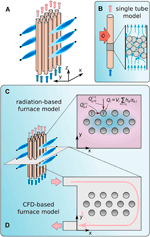FIGURE 1. Sketch of a side-fired tube bundle furnace (A) and three different models: the single tube model (B), the radiation-based furnace model (C), and the CFD-based furnace model (D).

In order to identify optimal tube bundle arrangements that include parameters such as optimal tube diameters, their placement and corresponding flue gas flows, an effective reduction of the full furnace complexity is essential in a way that the attained furnace simulations become computationally manageable at reasonable cost as shown in Figures 1B–D. For this reason the three-dimensional reforming furnace is decoupled, separating the inner tube processes from the furnace-side flow. This decoupling approach involve three distinct modeling steps.

In the first step, a single reforming tube is modeled as depicted in Figure 1B in order to capture transport, chemical conversion and axial dispersion in a single reforming tube. An axisymmetric pseudo-homogeneous model is used for a single synthesis tube that was presented and validated against industrial data earlier (Lao et al., 2016). In the consecutive step, the tube bundle arrangement in the furnace is investigated using cross-sectional models of the furnace—neglecting the axial coordinate—in order to address cross-sectional heat transport limitations and to identify the optimal bundle arrangement. This model decomposition is advantageous because shadow effects among tubes and associated flow patterns are expected to prevail over axial transport processes for side-fired furnace designs. At first, a purely radiation-based tube bundle furnace model is applied that was developed in a previous study (Liesche and Sundmacher, 2019) where temperature distribution must be assumed (Figure 1C). In the third step, the Navier-Stokes equations for the furnace cross section are solved using a computational fluid dynamics (CFD) model as depicted in Figure 1D. This procedure has the advantage that the fuel efficiency of a design can be evaluated based on the overall furnace energy balance.

These three modeling levels of increasing complexity are described in the next sections.

### 2.1. Single Tube Model

Reforming tubes in industry are designed as fixed bed reactors which in turn involve multi-scale processes ranging from molecular reaction steps to internal diffusion in catalyst pellets up to the scale of the overall reforming tube. The modeling of this level of detail, however, is not required in the context of the overall furnace design. Instead of small-scale phenomena that affect for example the dynamics of a reformer tube, the overall performance of a single tube is of interest. Therefore, the focus is on the impact of variations of the boundary conditions of a single reformer tube such as tube wall surface area and tube wall temperature. For this purpose the pseudo-homogeneous reforming tube model by Lao et al. is adopted. Here, the internal tube flow is modeled in an axisymmetric two-dimensional domain with a porous medium to reach pseudo-homogeneity which means that no local distinction between catalyst particles and continuous phase is made.

Using the single reformer tube model simulations are performed in order to deliver suitable boundary conditions for the cross-sectional furnace radiation-based and CFD models that are introduced below. These simulations are required to relate the tube surface temperature to the heat flux into the reforming tube. Moreover, these simulations are also used to quantify the reformer performance in terms of product yields and space-time yields.

The three chemical reactions—reforming, total reforming and water gas shift—of Eq. 1 are modeled using the reaction kinetic model of Xu and Froment that is frequently used in the modeling of reforming and methanation reactions (Xu and Froment, 1989a; Xu and Froment, 1989b; Bremer et al., 2017; Liesche and Sundmacher, 2018b). The rate expressions are multiplied with an effectiveness factor of 0.1 (Wesenberg and Svendsen, 2007), typical for steam reformer operation.

#### 2.1.1. Meshing

The tube is discretized using a sparse mesh based on the pseudo-homogeneous and axisymmetric model assumptions. Lengthwise, 1,000 elements are distributed equidistantly, while 10 elements in radial direction fill the domain. In radial direction, the elements are distributed hyperbolically where the first element height is set to 4.0 m $×{10}^{-4}$. A similar mesh size is reported in literature (Lao et al., 2016). Besides, variations of the meshing at this level of resolution have no significant impact on the results of the single reformer tube (see Supplementary Material). The simulations are carried out using the commercial CFD solver StarCCM + V13.02 (Siemens PLM).

#### 2.1.2. Single Tube Parameters

The gas composition at the inlet of a single reforming tube is a mixture of the gases CH4, H2O, CO, H2, and CO2 with the molar fractions of 0.2487, 0.7377, 0.0001, and 0.0117, respectively. The synthesis gas with a mass flow of and a temperature of ${T}_{in}=887\text{\hspace{0.17em}}\text{K}$ is entering the tube with an imposed parabolic inflow profile at the pressure of $p=3038\text{\hspace{0.17em}}\text{KPa}$.

The geometry of the single reforming tube reflects tube dimensions that are found in industrial steam reformers (Reimert et al., 2000; Lao et al., 2016). Its length is 12.5 m and its diameter is 12.6 cm (Lao et al., 2016). In order to study the impact of the tube geometry on the tube bundle performance, different radii of reforming tubes are investigated. Variations of the single reforming tube diameter has two direct consequences: increasing the diameter leads to increased radial transport resistance inside the tube. At the same time, the tube wall surface is increased, enhancing the heat transfer with the flue gas in the furnace. This impact is included in the study below when varying the reforming tube radius. The reference radius of 0.063 m is first considered; then, the radii are varied.

In addition, the assumed temperature profile at the wall by Lao et al. is replaced by a constant wall temperature, as Figure 2 illustrates. State-of-the-art steam reformer tubes sustain temperatures up to approximately 1,200 K (Reimert et al., 2000). In this numerical study of the single tube, temperatures up to 1,800 K are tolerated even though such high temperatures are presently considered infeasible. This is due to the fact that the single tube simulations serve as boundary conditions for the furnace simulations and numerical instabilities relating to a too narrowly defined wall temperature interval need to be avoided.

FIGURE 2

In summary, the surface wall temperature Twall, and reforming tube radii r are varied within the following range:

$Twall∈800,850,…,1800 Kr∈0.01,0.02,…,0.08 m∪0.063 m(2)$

For each possible tuple (${T}_{\text{wall}}$,r) stated in Eq. 2 a separate simulation is carried out. All other boundary conditions remain constant.

Based on the single reforming tube simulations, a radiation-based furnace model is adopted for the application of steam reforming (Liesche and Sundmacher, 2019). This two-dimensional furnace model is based on two fundamental assumptions for the furnace: convective heat transfer is neglected and two flue gas temperatures—within the bundle and in between the tube bundle and the furnace walls—are required. These assumptions enable an elegant formulation of the tube energy balances while maintaining the most significant features for the tube bundle design as shown in the results. The radiation-based model contains energy balances for each individual tube, as illustrated in Figure 1C: the heat of reaction inside the ith tube ${\stackrel{˙}{Q}}_{i}$ is supplied by radiative heat exchange with hot flue gas between the tube and furnace walls ${\stackrel{˙}{Q}}_{\text{g},iw}^{\left(r\right)}$, and by radiative heat exchange with the gas between the tubes ${\stackrel{˙}{Q}}_{\text{g},ij}^{\left(r\right)}$. The index $\text{g}$ denotes the flue gas. The distinction between wall-facing and tube-facing radiative heat flows is made because flue gas temperatures close to walls and burner inlets are assumed higher than between tubes, as previously observed in CFD studies of reformers (Zheng et al., 2010). Scattering in the furnace domain can be neglected due to the small path lengths compared to atmospheric radiation calculations. Nonetheless, it is important to take the flue gas radiation into account because it is the principle source of energy for any furnace application. The energy balance for each tube i is therefore formulated as

$∑w∈NwQ˙g,iw(r)+∑j∈Ntj≠iQ˙g,ij(r)+Qi=0 ∀i∈Nt(3)$

where ${\mathcal{N}}_{w}$ is the set of the bounding walls of the furnace and ${\mathcal{N}}_{t}$ is the set of all tubes. The heat of reaction Qi is obtained from the single tube model in dependence of the tube wall temperature. The radiative heat flows in the single tube energy balance 4 are calculated as

$Q˙g,iw(r)=1Rg,iwσ(Tg,w4−T(w),i4)(4a)$
$Q˙g,ij(r)=1Rg,ijσ(Tg,j4−T(w),i4)(4b)$

where ${T}_{\left(\text{w}\right),i}$ denotes the surface temperature of tube i and $\sigma$ the Stefan-Boltzmann constant. The analogy of radiative heat transfer to electrical circuit determination is used (Specht, 2017): the current corresponds to the radiative heat flow. For example the heat flow from the flue gas to tube i is given as the potential difference of the black body emissivity divided by the total resistance. ${R}_{\text{g},iw}$ and ${R}_{\text{g},ij}$ repre ue gas, wall w and tube i, and between flue gas and tubes i and j, respectively. The two resistances of Eq. 4 are formulated as

$Rg,iw=1φiwAiεg(2−εg)+1−εiεiφiwAiRg,ij=1φijAiεg(2−εg)+1−εiεiφijAi(5)$

where equal areas ${A}_{i}$ of all tubes $i\in {\mathcal{N}}_{t}$ are assumed, and ${\mathit{\epsilon }}_{i}$ is the tube surface emissivity. It is important to note that wall emissivities are irrelevant if near-adiabatic walls can be assumed. A detailed derivation of the resistance expressions can be found in Liesche and Sundmacher (2019).

The gas emissivity ${\mathit{\epsilon }}_{\text{g}}$ is calculated using Lambert-Beer’s law (VDI-Gesellschaft Verfahrenstechnik und Chemieingenieurwesen, 2013):

$εg=1−exp(−∑ακαpαΔs)(6)$

In this equation ${\kappa }_{\alpha }$ and ${p}_{\alpha }$ represent the temperature-dependent absorption coefficient and the partial pressure of component α. The thickness of the gas layer is denoted as ${\text{Δ}}_{s}$. Planck mean absorption coefficients are used as gray-gas absorption coefficients (Zhang and Modest, 2002; Liesche and Sundmacher, 2018a).

The parameters φiw and φij denote view factors between the ith tube and wth wall as well as with another tube j. In general, the view factor φij relates the overall heat flux of surface i to the heat flux between surfaces i and j (Modest, 2013):

$dQ˙ij=φijdQ˙i(7)$

All surfaces are assumed to be homogeneous and diffuse emitters which means that the directional distribution of the radiative intensity leaving the surface is described by Lambert’s law (Modest, 2013). Consequently,

$φijAi≡φjiAj(8)$

and for inter-tube view factors with equal tube surface areas

$φij≡φji(9)$

applies. The challenge of the view factor determination in a tube bundle is the correct description of shadowing effects between tubes. This is done using hyperbolic tangent functions as switching functions in the interval $\varphi \in \left[0,2\right)$ where the viewing angle between two tubes is described by the product of two functions:

$f¯ij=ξ1tanh(ϕ−θ¯ijξ2)+ξ3f¯ij=1−(ξ1tanh(ϕ−θ¯ijξ2)+ξ3)(10)$

where ${\underset{¯}{\theta }}_{i}j\left({x}_{i},{y}_{i},{x}_{j},{y}_{j}\right)$ and ${\overline{\theta }}_{ij}\left({x}_{i},{y}_{i},{x}_{j},{y}_{j}\right)$ represent the lower and upper bound of the view angle of i toward j that are dependent on the positions of both tubes. The two parameters ${\xi }_{1}$ and ${\xi }_{3}$ are set to 0.5 to ensure that the visual angle function values remain in $\left[0,1\right]$ and that the function attains unity if tube j is visible from position (${x}_{i}$, ${y}_{i}$), and zero if it is not visible. The third parameter ${\xi }_{2}$ is set as small as possible because it determines the steepness of the function switch: the steeper the function, the more accurate the view factor representation, but also the harder the integration of the stiffer view factor function. The angular function describing the visual angle from i to j is then given by the product of the lower and upper bound functions

$fij=f¯ijf¯ij(11)$

Subsequently, the view factor φij is determined as the integration of this function over the entire angular space divided by $2\pi$

$φij=12π∫​02πfijdϕ(12)$

In order to quantify shadowing it is irrelevant for the total inter-tube view factor if tube i is shaded by tube j or vice versa. Instead, the total inter-tube view factors of each tube i and of the overall bundle are of interest. Using the formulation shown in Eq. 11, the total visual angle of tubes j and k from i is given as the sum of the functions subtracted by their product

$fijk=fij+fik−fijfik(13)$

This method of view factor calculation between tubes is compared with the crossed-strings method (Modest, 2013) vs. the ratio $h:={l}_{ij}/r$ in Figure 3. In this ratio, r is the radius of tube and ${l}_{ij}$ the distance between tubes i and j. At the minimum allowed tube distance of $h=3$ the error of the proposed view factor calculation methodology accounts for 6.2%. At $h=4$, the error drops already below 4.0% making this deviation negligible. In the crossed-strings method, the diagonals and sides of a hypothetical trapezoid need to be calculated, which is valid as long as the diagonals form straight lines (Modest, 2013). However, the shadowing of tubes by a multitude of other tubes with flexible positions is contrary to this assumption and areas of exchange would have to be recalculated for every new positioning in order to guarantee straight diagonals. As a consequence, the proposed method of view factor calculation is preferred over the crossed-strings method despite its small deviations for densely packed tube arrangements.

FIGURE 3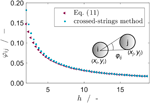FIGURE 3. View factors between tube i and j: ${\phi }_{ij}$ calculated using Eq. 12 (red triangles) and compared with the crossed-strings method (blue circles) vs. the distance ratio $h:={l}_{ij}/r$.

### 2.3. CFD-Based Furnace Model

In addition to the radiation-based model, a CFD-based model of the two dimensional flow domain is formulated as illustrated in Figure 1D. The consideration of a two-dimensional cross section instead of the full-scale furnace where entry and exhaust regions are neglected is self-evident: it does not match the predictive power of a simulation which fully resolves turbulence, kinetics, and radiation. However, this approach enables the simulation of multiple design configurations at comparatively low computational cost of each individual simulation. Thus, furnace configurations can be compared and the best design out of the design search space is identified as opposed to an in-depth modeling of a single scenario (Tran et al., 2017).

The target of the CFD-based furnace model that is depicted in Figure 1D is the identification of the best tube bundle arrangement in the furnace. When considering a variable and large number of tubes, a full optimization study is in principle possible but it requires a huge number of CFD simulations (Daróczy et al., 2014). In that study, we considered the identification of optimal tube bundle heat exchangers for liquid flow and within a narrow temperature window as compared to the high temperature application of a tube bundle furnace where additional models such as radiative heat transfer need to be considered. An optimization along the lines of Daróczy et al. would lead to unacceptably high computational costs here. As an alternative, a simulation-based optimization approach based on current tube bundle furnace designs that considers selected geometrical distributions is adequate to identify the optimal tube arrangement.

#### 2.3.1. Parametrization of Geometry

The position of a reformer tube of circular cross section is uniquely described by the coordinates of its center resulting in two parameters per tube. This parameter space is reduced even further to one distance factor per arrangement by assuming typical industrial arrangements such as a single line tube arrangement of top-fired reformers and aligned, staggered and rectangular arrangements that are used in BMA reactors for HCN synthesis. Besides, the tube radius must be specified. The resulting four different types of bundle arrangements that are considered in this study are illustrated in Figure 4.

FIGURE 4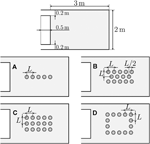FIGURE 4. Schematic representation of the furnace geometry and four canonical tube arrangements assessed in the parameter study. Configurations: line (A), staggered (B), aligned (C), and rectangle (D).

The furnace geometry for all bundle designs is fixed as a rectangular cross section with dimensions 2 $×$ 3 m excluding the inlet and outlet channels. These channels are 0.5 m in length and 0.2 m wide. In all configuration templates shown in Figure 4, the parameter L specifies a characteristic distance between the respective set of tubes. All tube bundle arrangements are centered within the furnace.

The configuration A is a linear configuration with five tubes. They are equidistantly distributed with the inter-tube distance of L. This configuration is a common arrangement for real furnaces, for example used by Tran et al. The free volume around this tube arrangement is large, resulting in high flue gas emissivities.

The configuration denoted as B stems from the arrangement that is typically applied in BMA reactors which were investigated in a separate study (Liesche and Sundmacher, 2019). It consists of 14 tubes, arranged in two rows of five enclosing one row of four tubes. Within each row, the tubes are again equidistantly distributed. The middle row is shifted by $L/2$ to get a staggered arrangement.

The configuration denoted as C is similar to the previous configuration. However, it contains 15 tubes which are equally distributed in both directions without staggering between the layers. The intention of this configuration is to investigate shadowing effects in an arrangement with many local symmetries.

The last configuration denoted as D is arranged in a rectangular shape with a hollow core. It contains 14 tubes and is a super-structure of A.

#### 2.3.2. Parameter Selection for the Design Study

For all four tube bundle configurations, two design parameters remain: the characteristic distance L, and the inner tube radius r. The latter must obviously be kept small enough compared to L in order to avoid tube collisions. This small number of parameter enables a systematic parameter study. Results for inner tube radii r are presented for three different values—a typical industrially applied radius of 0.063 m, and one smaller and one larger radius of 0.040 and 0.080 m, respectively. A constant tube wall thickness of 0.01 m is assumed.

The second parameter from the geometrical parameterization, L, is obtained as the product of the distance factor h and the reference tube radius $r=0.063\text{\hspace{0.17em}}\text{m}$, leading to:

$L=h D0.063=2h(0.063 m+0.01 m)(14)$

The following values for the distance factor h are considered in this study:

$h∈[1.25,1.5,2.0,2.25,2.375,2.5,2.75,3.0,3.5,4.0,4.25](15)$

These values include industrially applied inter-tube distances (Reimert et al., 2000; Lao et al., 2016; Tran et al., 2017). Besides, cases ranging from tightly-packed bundles up to tube bundle configurations where the outer tubes are close to the inner furnace walls are considered.

In addition to these geometrical parameters, the flue gas mass flow is varied on the basis of individual tubes in order to enable a fair comparison of the four design types with their varying tube numbers.

The upper mass flow value limits the maximum inflow velocity of flue gas to values that are experienced in furnaces of approximately 15 m s−1 (Zheng et al., 2010). The total flue gas mass flow $\stackrel{˙}{m}$ is hence proportional to the number of tubes N, and varies among the four different canonical configurations.

For each possible parameter combination (r,${\stackrel{˙}{m}}_{/t}$,h) an individual CFD simulation is carried out with the setup described in the next section. This results in a total number of 1,008 individual CFD simulations.

#### 2.3.3. CFD Setup

The large flow domain, relatively high velocities, and low viscosity of the flue gas make the flow inside such a furnace inherently turbulent. Turbulence is modeled in CFD using the Reynolds-Averaged Navier-Stokes (RANS) equations. The well-established, two-equation realizable k-ε model is used to model turbulent effects. Wall boundary layers are represented with a two-layer wall function, which blends between a fully resolved boundary layer and a wall model. Inflow turbulence intensity is assumed in a standard manner to be $1\text{%}$. The turbulent viscosity ratio is assumed as 10. Due to the large number of simulations required for this study, solely steady-state results are considered, so that the follow-up analysis neglects any possible transient effects.

In the real process, fuel combustion takes place within the furnace to heat up the tube bundles. Similar to the single reforming tube, however, resolving of the full-scale microscopic phenomena on the furnace is not within the scope of this project because it is not helpful for the overall comparative evaluation of bundle arrangements. In addition, resolving a high level of detail is limited by the involved high computational cost (Hilbert et al., 2004). As a consequence, the combustion and flame structure inside the furnace are replaced with an nonreactive hot flue gas that stems from the complete combustion of methane. The flue gas is modeled as an ideal gas mixture of CO2, H2O, O2, and N2 with molar fractions of 0.05, 0.10, 0.10, and 0.75, respectively, that enters the furnace domain at 1,800 K.

Similar to the single tube and radiation-based model, a gray participating medium is adequate to account for radiative heat transfer and scattering effects are neglected. The discrete ordinate method (DOM), is selected as modeling approach for radiation. The furnace walls are considered adiabatic, gray, homogeneous and diffuse emitters. The wall emissivities ${\mathit{\epsilon }}_{{i}_{\text{w}}}$ are set to 0.65 and the reforming tube surface emissivities ${\mathit{\epsilon }}_{i}$ are set to 0.8 (Tran et al., 2017).

The absorption coefficient of the flue gas ${\kappa }_{\text{g}}$ is independent of pure components and thus depends only on H2O and CO2. The absorption coefficient of the flue gas is computed with

$κg=ppref(xH2OκH2O+κCO2 κCO2)(17)$

The ratio $\frac{p}{{p}_{ref}}$ is the ratio of the local pressure to the reference pressure, while x is the mole fraction of the respective component. The absorption coefficients of the pure substances are temperature-dependent and they are calculated using the polynomial function:

$κi=∑j=013aij Tj(18)$

The constants ${a}_{ij}$ can be found in the Supplementary Material.

The endothermic reforming reaction inside the tubes is driven by the heat flux into the reforming tubes. The temperature-dependent heat flux that is obtained from single tube simulations is thus served as a boundary condition for the furnace simulations. The thermal boundary layer on the tube surfaces coupled to convection is derived from the evolution of the turbulent boundary layer along all walls. The wall thickness of the tubes is taken into account when computing the heat flux to the tubes within the bundle, using standard theory for steady heat flux through a pipe wall.

The outlet of the furnace cross section is a zero-gradient pressure outlet boundary. To increase numerical stability, temporary backflow conditions in case of occurring backflow are tolerated. Similar to the simulations of the single reforming tubes, all simulations are carried out with the CFD solver StarCCM + V13.02. In addition, the furnace simulations are automatized using a Java program and StarCCM+‘s application programming interface (API). The computations were partly performed on the high performance computing cluster “Mechthild” of the Max Planck Institute for Dynamics of Complex Technical Systems.

#### 2.3.4. Meshing of the Furnace Domain

For each individual simulation a polyhedral mesh is generated for the whole domain. Near the walls, 35 prismatic layers are used to resolve the flow and temperature boundary layer, with a growth factor of 1.2. In order to scrutinize the validity of the simulations, a mesh-dependency study is first carried out. The results of this mesh study are exemplified for the total heat flux into all tubes in the Supplementary Material. The total heat flux ${\stackrel{˙}{Q}}_{\text{Σ}}$ is computed by the sum of heat fluxes of the N tubes of a tube bundle configuration:

$Q˙Σ=∑i=1NQ˙i(19)$

A mesh size of around 800,000 elements is selected because it is a good compromise between duration of computation and accuracy for the furnace scenario. The deviation to a mesh with twice the number of cells is very low with 0.28%. The total number of cells is kept constant across all furnace simulations.

Using some individual adaptation for few scenarios, 975 simulations out of the 1,008 converged based on the described CFD setup and mesh topology.

## 3. Results

The results section is structured according to the hierarchical furnace design procedure that is illustrated in Figure 1. Initially, key performance characteristics are defined based on single reforming tube simulations. At the same time, the correlations of these performance numbers with heat flux to the reforming tubes are highlighted. Consecutively, the prevalence of radiative heat transfer is analyzed for the proposed furnace design space and the applicability of the radiation-based furnace model for quick comparative decision-making is illustrated. The final part of the results section identifies the most promising design candidate among the investigated samples based on the performance criteria of the single-tube simulations as well as energy efficiency.

### 3.1. Single Reformer Tube

Two key design parameters of a single reforming tubes are identified as the tube radius and the temperature of the tube wall. The impact of both parameters is illustrated in Figure 1. The wall heat flux ${\stackrel{˙}{Q}}_{\text{wall}}$ vs. wall temperature ${T}_{\text{wall}}$ is depicted on the right for a wide range of tube radii. The heat fluxes of all tube radii increase more steeply for temperatures below 1,200 K compared with higher temperatures. The reason for this behavior is illustrated on the left hand side of Figure 5; the yield of the target product CO vs. the wall temperature is shown here. At temperatures above 1,200 K, the major share of the reactants has already been converted to products and the slope of the yield decreases.

FIGURE 5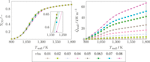FIGURE 5 . Yield of CO, YCO of a single reforming tube, and wall heat flux ${\stackrel{˙}{Q}}_{\text{wall}}$ per meter of tube, as result of different wall temperatures ${T}_{\text{wall}}$ and inner tube radii r. The left graph contains a zoomed view of the curves at 1,200 K as overlayed subplot.

The production of synthesis gas—a mixture of H2 and CO—is limited by the fact that a certain share of CO2 is simultaneously formed due to the chemical equilibrium. For this reason, the yield of CO, ${Y}_{\text{CO}}$, is selected as a performance indicator for each individual tube. The product yield is obtained as the product of the conversion of CH4 and the selectivity toward CO (Davis and Davis, 2012):

$YCO=XCH4 SCO(20)$

The conversion of the reactant methane CH is calculated between inlet and outlet of a single reforming tube as:

$XCH4=1−n˙CH4,outn˙CH4,in$

Selectivity toward CO is calculated as:

$SCO=n˙CO,out−n˙CO,inn˙CO,out−n˙CO,in+n˙CO2,out−n˙CO2,in$

Whereas the yield is a measure for product quality, the space time yield STYi is introduced as a measure of the product quantity that is obtained. For this purpose the product molar flow is related to the catalyst volume inside of the reactor. The latter increases quadratically with the tube radii, which is why it is considered for the variation of tube radii. The space-time yield for the target product $\text{CO}$ is defined as

$STYiCO:=n˙CO,out−n˙CO,inVcat$

An additional aspect is visible from both Figure 5: Almost equal yields are attained irrespective of the reforming tube radii and despite an increase in radial transport resistance with larger radii. Nonetheless, there are differences in product yield for the decisive temperature range between 1,050 and 1,300 K. It is also important to highlight that a significantly larger amount of heat is transferred to the tubes with larger tube radii as opposed to smaller ones. At the same time, the utilization of smaller tubes leads to higher pressure drops for equal mass flows in each tube. All these results show that there is a trade-off between smaller and larger tube radii on the reforming side: smaller tube radii are beneficial for high yields but suffer from higher pressure drops and vice versa for large tube radii.

### 3.2. Prevalence of Radiative Heat Transfer

The prevalence of radiative heat transfer can be estimated with the dimensionless Boltzmann number B which is the convection-to-radiation ratio:

$BO:=ρcpνn2σT3(21)$

In this equation $\rho$, ${c}_{p}$ and ν denote the gas density, heat capacity and velocity, whereas n, σ and T represent the refractive index of the fluid, the Stefan-Boltzmann constant and the temperature. For the furnace flue gas composition specified in Section 2.3.3 and approximating the refractive index with unity, the obtained values of B as a function of temperature are shown in Figure 6.

FIGURE 6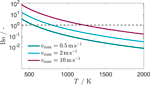FIGURE 6. Boltzmann number B for three velocities ${\nu }_{\text{max}}\in \left\{0.5,2,10\right\}$ ms.

For low velocities of $\nu =0.5\text{\hspace{0.17em}}\text{m}\text{\hspace{0.17em}}\text{s}$, radiative heat transfer prevails; the Boltzmann number is below the dotted line in Figure 6 already for temperatures as low as 600 K. At $\nu =2\text{\hspace{0.17em}}\text{m}\text{\hspace{0.17em}}\text{s}$ and $\nu =10\text{\hspace{0.17em}}\text{m}\text{\hspace{0.17em}}\text{s}$ this tipping point temperature increases to 800 and 1,200 K, respectively, because convective heat transfer is more relevant at those high gas velocities. A selection of furnace simulations for rectangular scenarios D is presented in Figure 7 where the velocities of the scenario are shown in the left column and the corresponding temperature profiles in the right column.

FIGURE 7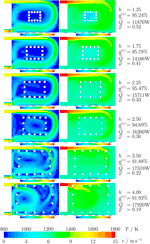FIGURE 7. Velocity magnitude (left column) and temperature fields (right column) for the configuration D at different distance factors with an inner tube radius of $r=0.063\text{\hspace{0.17em}}\text{m}$, and a flue gas mass flow of ${\stackrel{˙}{m}}_{/t}=0.03\text{\hspace{0.17em}}\text{kg/s/tube}$.

The velocity magnitudes vary from close to zero between tubes up to 15 ms at the flue gas inlet in the top left corner. This velocity distribution illustrates that tubes along top and right-hand furnace walls are closest to the main flow of hot gases. When h is large, these tubes are directly exposed to the main stream. Looking at the velocities of the top four simulations, the following behavior is observed: In the vicinity of the tube arrangement, velocities drop below $\nu =2\text{\hspace{0.17em}}\text{m}\text{\hspace{0.17em}}\text{s}$. In the center of the rectangular enclosure of the reforming tubes, there is almost no gas flow at all and flue gas velocities tend toward zero. For the bottom two scenarios with distance factors of $h=3.5$ and $h=4.0$, the inter-tube distance is sufficiently large that the tubes obstruct the main flow and a different velocity profile is formed where significant flow across the center of the rectangular arrangement occurs. It is expected from these scenarios that convection is by far negligible for the distance factors of $h\le 3.5$ and that its importance increases beyond that for rectangular arrangements.

In order to quantify this assumption for all furnace design scenarios, a mean radiative heat flux ratio ${q}^{\left(r\right)}$ is introduced as the ratio of the radiative flux ${\stackrel{˙}{Q}}_{i}$ and the total heat flux ${\stackrel{˙}{Q}}_{\text{Σ}}$:

$q(r)=1Q˙Σ∑i=1NQ˙i(r)(22)$

The radiative heat flux ratio ${q}^{\left(r\right)}$ is illustrated vs. the mean of the heat flux that is transferred to a bundle arrangement in Figure 8. It is immediately evident, that radiative heat transfer dominates for all investigated bundle arrangements. Irrespective of the size and arrangements of the tubes, radiative heat flux accounts for at least 88% of the total heat flux into the reforming tubes. The remaining fraction is conveyed via convective heat transfer. All line arrangements A are not exposed to the convective flue gas flow near the furnace walls even if the distance factor is set to its maximum value. Therefore, radiative heat flux which is denoted with magenta stars in Figure 8 accounts for at least 95% for all line arrangements. This observation is in agreement with literature on heat transfer in industrial-scale steam reformers (Yu et al., 2006).

FIGURE 8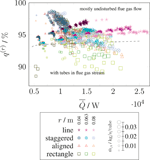FIGURE 8. Radiative heat flux ratio ${q}^{\left(r\right)}$ in relation to the mean heat flux per tube $\overline{\stackrel{˙}{Q}}$. The symbol (and its color family) indicates the configuration of the data point. Shades of the respective color indicate the inner tube radius r. The symbol size indicates the mass flow rate ${\stackrel{˙}{m}}_{/t}$ of flue gas per reforming tube.

A black line in Figure 8 separates scenarios where tubes are placed near or within the flue gas flow (bottom) to scenarios where the tubes remain at a distance from the furnace walls (top). In particular for the rectangular arrangement D that is illustrated with squares in Figure 8 convection plays a more important role especially for the largest tube radii. The reason for the importance of convection for rectangular arrangements is already visible in Figure 4. Whereas the line arrangement (A) does not expand in transverse direction for larger L, staggered and aligned arrangements (B, C) stretch with $2L$ in transverse direction. For rectangular arrangements (D), however, a single arrangement stretches across $3L$. Irrespective of diameter and distance factor, rectangular arrangements are thus closer to the flue gas flow, which is also shown in Figure 7. A slightly disturbed hot gas stream (Figure 7, $h=3.5$ and $h=4.0$) increases the overall heat flux at first. In extreme cases of $h\ge 4$, however, the tubes block the main flue gas stream, which strongly impacts the overall flow structure and leads to lower overall performance.

In addition to the geometrical parameters, Figure 8 demonstrates the impact of increasing flue gas mass flows. Generally, convective heat transfer increases with the flue gas mass flow. If high flue gas mass flows, large tube radii, and large distance factors occur in the same simulation, convection conveys 5–10% of the total heat flux, and even up to 12% in some configurations.

### 3.3. Applicability of the Radiation-Based Model

The applicability of the simplified radiation-based model for tube bundle design of furnace that is described in Section 2.2 is now analyzed. Two assumptions are essential in order to apply this model: 1) the dominance of radiative heat transfer in the furnace and 2) the assumption that a relatively homogeneous temperature distribution in the furnace can be assumed with a higher temperature near the furnace walls compared to the furnace space in between the tubes. The first assumption has been justified in the previous section and the latter is illustrated in Figure 7 in the right column. The flue gas enters the domain with temperatures of 1,800 K. This temperature drops very rapidly to values in the range 1,300–1,500 K around the tubes, and later 1,100–1,200 K in-between tubes and in the center of the bundle arrangement if the tubes do not obstruct the flue gas flow. As a consequence, the resulting temperature distribution can be approximated using the two temperature domains of the radiation-based model (Liesche and Sundmacher, 2019).

Two parameters that influence furnace bundle design were identified previously (Liesche and Sundmacher, 2019): the flue gas emissivity and the view factors. Whereas the impact of flue gas emissivities cannot be directly observed in the CFD simulations due to the overlay of convection near furnace walls the view factors for all furnace simulations can be observed. The mean of the inter-tube view factors for any given furnace simulation is defined as

$φ¯=1N∑i=1N∑j=1Nφij(23)$

Results for all furnace simulations are illustrated in Figure 9.

FIGURE 9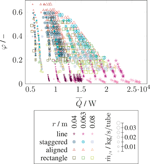FIGURE 9. Average view factor $\overline{\phi }$ vs. mean heat flux $\overline{\stackrel{˙}{Q}}$. The symbol (and its color family) indicates the configuration of the data point. Shades of the respective color indicate the inner tube radius r. Symbol size indicates the mass flow rate ${\stackrel{˙}{m}}_{/t}$ of flue gas per reforming tube.

For almost all cases, a smaller $\overline{\phi }$ correlates with a larger $\overline{\stackrel{˙}{Q}}$. This confirms the expectations because a smaller view factor corresponds to cases with higher radiation. It can also be observed that configurations with a larger radius lead to a higher mean heat flux and dependence of mean heat flux on the average view factor is more pronounced for higher flue gas flows (larger symbols). Line configurations (A) have the lowest view factors compared to the other geometrical arrangements. This is the result of low number of tubes in the line arrangement that do not include any shadowing in the transverse direction which in turn is a result of the fixed furnace size. Naturally, the total and also average view factors are larger if the same space is populated with more tubes as is the case for all other arrangements B, C and D.

FIGURE 10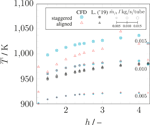FIGURE 10. Mean tube surface temperature of all tubes in the bundle $\overline{T}$ vs. distance factor h for staggered and aligned arrangements. Results from the radiation-based model (Liesche and Sundmacher, 2019) are marked with black symbols of the respective staggered and aligned arrangements.

Both furnace models are compared for different tube diameters including the standard deviations of tube surface temperatures in Figure 11. As before, there is a qualitative agreement of both models but the tube surface temperatures vary more strongly in the CFD-based model. Similar to Figure 10 this effect stems from the approximations of the temperature field. The mean surface temperatures increase with increasing distance h for all scenarios and all models. A second important aspect are the larger standard deviations across the tube bundle for the CFD-based model. The tubes at the boundaries of the staggered bundle are affected by convection whereas the inner tubes see nearly no convection at all. That explains the stronger variations of tube temperatures across the tube bundle. In addition, the minimum of standard deviations occurs at smaller values of the distance factor h compared to the radiation-based model because the impact of convection increases with h in the CFD-based model. As a consequence, variations in the tube surface temperatures should be analyzed preferably with the CFD-based model.

FIGURE 11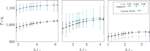FIGURE 11. (A) Mean tube surface temperature $\overline{T}$ vs. distance factor h for staggered arrangements (B) based on the radiation model (black) and CFD model (blue) for three different tube radii. Error bars show the standard deviation of the respective data set.

In summary, the radiation-based model is fully sufficient for a fast comparison of different furnace geometries in terms of overall performance. The CFD-based model should be preferred in situations where the absolute tube surface temperature values and variations across the tube bundle are of primary interest. In addition, the energy efficiency of a bundle design can only be identified using the CFD-based model because the overall energy balance is included for the furnace flue gas flow.

### 3.4. Identification of the Optimal Tube Bundle Arrangement

The last part of the previous section showed that variations of tube temperatures in a bundle can be assessed with more detail using the CFD-based model. Therefore, the optimal bundle arrangement among the case studies that were analyzed in this study is identified using the CFD-based model. The optimal scenario is identified using the three design parameters that were identified in section 2.3 as radius r, distance factor h and flue gas massflow ${\stackrel{˙}{m}}_{/t}$. The mean temperature of all tubes in an arrangement vs. the mean heat flux is illustrated in Figure 12. In any industrial furnace it is undesired that tubes are exposed directly to the hot flue gas stream because that leads to large temperature gradients and temperature fluctuations along the tube walls, resulting in high thermal stresses and overall non-homogeneous process conditions. For this reason, minimum and maximum temperatures of each simulation are included as vertical pseudo-error bars.

FIGURE 12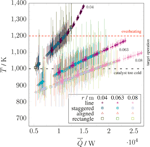FIGURE 12. Mean wall temperature $\overline{T}$ vs. mean heat flux $\overline{\stackrel{˙}{Q}}$. Minimum and maximum temperatures of each simulation are included as pseudo-error bars. Upper operation limit of tubes (red dotted line) and lower operation limit (black dotted line) are included. Symbols (and associated color family) indicate the geometrical configuration corresponding to the data point. Shades of the respective color indicate the inner tube radius r.

In A configurations, mean temperatures and mean heat transfer are higher than in all the other configurations. Moreover, the minimum and maximum temperatures for A are very close to $\overline{T}$ indicating a very homogeneous temperature distribution which is beneficial to ensure high process quality. In the remaining three configurations, especially in D, the minimum and maximum temperatures differ by a wide amount around the mean temperature. This is due to the transverse placement of the tubes in the furnace which in turn causes some shadowing as well as pronounced convection effects.

In addition, a feasibility window based on industrial steam reformers is indicated using the red (upper operating temperature) and black (lower operating temperature) dotted lines (Reimert et al., 2000). If the temperature is too low, the conversion is too low or no firing of the catalyst occurs. Vice versa, overheating and material degradation occur at high temperatures. It is thus desirable to attain the region bounded by these two lines on the far right where a high mean heat flux is achieved. For small tube radii (dark-shaded symbols) overheating may be encountered and for large tube radii (bright shaded symbols) temperatures are lower than the desired temperature except for line arrangements where the maximum mean heat flux is achieved. All flue gas mass flows are included in Figure 12 but not highlighted separately in order to maintain good readability of the figure. It is nevertheless visible that results of an arrangement type and radius seem to cluster: this is due to the flue gas mass flows where large mass flows are to be found on the upper right end and low mass flows toward the bottom left.

For the tube radius that is frequently found in industry (0.063 m) any of the four arrangements can be found in the optimal temperature interval. Yet, some configurations have strong deviations within the bundle; the line arrangement (A) is again superior over the other tube bundle arrangements.

The impact of the three decisive furnace parameters on the space-time yield of $\text{CO}$ is illustrated in Figure 13. There, STYCO is shown vs. the distance factor h. Each configuration is shown in a separate subplot; three radii are shown and the symbol size indicates the flue gas mass flow.

FIGURE 13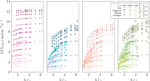FIGURE 13. The space-time yield STYiCO as a function of the distance factor h—shown for each configuration individually. The symbol (and its color family) indicates the configuration of the data point. Shades of the respective color indicate the inner tube radius r. Symbol size indicates the mass flow rate ${\stackrel{˙}{m}}_{/t}$ of flue gas per reforming tube.

This graph shows that smaller tube radii combined with high flue gas mass flows perform better in terms of space-time yield than larger tubes. Secondly, the space-time yield increases with the distance factor until reaching a plateau. This effect is emphasized more strongly for configurations B, C and D. For the considered furnace geometry, the minimal distance between the tubes h is approximately three to reach the plateau. Thirdly, the line configuration A outperforms the other arrangements. In addition, STYCO decreases slightly above a distance factor of four for the inner tube radius of 0.063 m. This effect was explained above where the tubes obstruct the flue gas flow for large distance factors h. This effect is not visible for the smallest tube radius of 0.04 m.

One aspect remains to be analyzed: the energy efficiency of the furnace designs. For this purpose, the total heat flux from the flue gas into the reforming per mass of flue gas (${\stackrel{˙}{Q}}_{\text{Σ}}^{\left(\stackrel{˙}{m}\right)}$) is used. With the total heat flux ${\stackrel{˙}{Q}}_{\text{Σ}}$, as defined in Eq. 19, ${\stackrel{˙}{Q}}_{\text{Σ}}^{\left(\stackrel{˙}{m}\right)}$ is computed as:

$Q˙Σ(m˙)=1m˙Q˙Σ(24)$

where $\stackrel{˙}{m}$ is the total mass flow of flue gas. That mass flow is the product of the per tube mass flow $\stackrel{˙}{m}/t$ and the number of tubes N. The mean heat flux into the reforming tubes is straight-forwardly computed by:

$Q˙¯=1NQ˙Σ(25)$

Accordingly, ${\stackrel{˙}{Q}}_{\text{Σ}}^{\left(\stackrel{˙}{m}\right)}$ vs. $\overline{\stackrel{˙}{Q}}$ is plotted in Figure 14. Equations 24 and 25 show that there is a linear dependency between both properties and a trade-off has to be made: for increasing ${\stackrel{˙}{m}}_{/t}$, ${\stackrel{˙}{Q}}_{\text{Σ}}^{\left(\stackrel{˙}{m}\right)}$ decreases for similar configurations. On the other side, the mean heat flux $\overline{\stackrel{˙}{Q}}$ increases with a higher flue gas inflow. This means, at low flue gas mass flows, a higher proportion of heat is transferred from the flue gas to the tubes. These cases have the highest energy efficiency but are limited in terms of total heat transferred.

FIGURE 14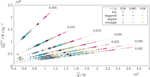FIGURE 14. Total heat flux ${\stackrel{˙}{Q}}_{\text{Σ}}^{\left(\stackrel{˙}{m}\right)}$ scaled by the mass flow of flue gas in relation to the mean heat flux per tube $\overline{\stackrel{˙}{Q}}$. The dashed lines with labels indicate configurations with equal flue gas inflow ${\stackrel{˙}{m}}_{/t}$ per tube in kg/s/tube. The symbol (and its color family) indicates the configuration of the data point. Shades of the respective color indicate the inner tube radius r.

Contrarily, a high flue gas inflow naturally has more transferable heat. The temperature of the flue gas decreases not as much for an equal amount of heat as in low flue gas mass flow scenarios. More heat and a higher temperature difference is left, which enables further heat to be transferred. Consequently, the flue gas exits the furnace at higher temperatures, which therefore reduces energy efficiency.

All design considerations of this section result in the following optimal tube bundle arrangement for the furnace under investigation. Line arrangements shall generally be preferred using a small tube radius of 0.04 m if the related pressure drop and coking are manageable. The inter-tube distance is of less importance for this line arrangement but a distance factor of $h\ge 3$ ensures a high performance. With respect to energy efficiency and product quantity (Figures 13 and 14) a mass flow per tube of represents an adequate intermediate value. Increasing the mass flow leads to marginal gains in STYi at the expense of lower energy efficiency of the design.

## 4. Conclusion

In summary, the targeted decoupling strategy for the simulation of the complex three-dimensional tube bundle furnace leads to the following results. Single reforming tube simulations for different tube radii and surface temperatures are made to obtain boundary conditions for furnace-scale simulations. Two models are proposed for the identification of optimal design on the furnace-scale—one based on radiative heat transfer only, and a CFD-based model. Modeling the furnace environment based entirely on radiative heat transfer is a good approximation and enables an effective comparison of tube bundle furnace arrangements, leading to a suitable tool for quick design comparison. Due to the abundance of industrial-scale steam reformers, however, the focus of future novel reactor developments lays in fine-tuned tube arrangements where standard deviation of wall temperatures in a bundle and fuel efficiency can be analyzed. Based on the furnace geometry and design space, the optimal design is to arrange the tubes in lines and to favor tube radii that are as small as possible for effective heat transfer and conversion inside of the tubes. Flue gas mass flows of ${\stackrel{˙}{m}}_{/t}=0.020\text{\hspace{0.17em}}{\text{kg/s}}^{-1}\text{/tube}$ represent a good balance between fuel efficiency and product yield but may vary depending on site-specific conditions. In addition, tube distance factors of $h\ge 3$ are recommendable for any tube bundle arrangement based on this systematic study.

Using the radiation-based design tool and CFD simulations, existing designs can be evaluated as well as novel designs identified. In a follow-up study the furnace geometry should be varied to ensure that the furnace space per tube does not vary significantly in order to avoid any bias originating from flue gas residence times in the furnace. The impact of oxygen-enriched combustion is another aspect that may be of interest for furnaces because higher molar fractions of the emissive gases CO and HO would enhance radiative heat transfer. Both aspects, however, can hardly be optimized without a specific plant context.

## Data Availability Statement

The datasets presented in this study can be found in online repositories. The names of the repository/repositories and accession number(s) can be found below: https://doi.org/10.5281/zenodo.3944732.

## Author Contributions

SE and GL wrote this article. SE created the CFD models, performed the simulations and its analysis. GL created radiation-based modeling and simulations and its analysis. KS, GJ, and DT supervised the study and rigorously revised the manuscript. All authors contributed to the article and approved the submitted version.

## Funding

This publication is supported by the EU-program ERDF (European Regional Development Fund) within the research center of dynamic systems (CDS).

## Conflict of Interest

The authors declare that the research was conducted in the absence of any commercial or financial relationships that could be construed as a potential conflict of interest.

## Acknowledgments

The author Sebastian Engel is affiliated with the International Max Planck Research School (IMPRS) for Advanced Methods in Process Systems Engineering (Magdeburg). We thank our students Adrian Krummnow and Saketh Bharadwaj for their contributions to this study.

## Supplementary Material

The Supplementary Material for this article can be found online at: https://www.frontiersin.org/articles/10.3389/fenrg.2020.583346/full#supplementary-material

## References

Bazzanella, A. M., and Ausfelder, F. (2017). Technology study: low carbon energy and feedstock for the European chemical industry. Frankfurt a.M., Germany: DECHEMA Gesellschaft für Chemische Technik und Biotechnologie e.V.

Bremer, J., Rätze, K. H. G., and Sundmacher, K. (2017). CO2 methanation: optimal start-up control of a fixed-bed reactor for power-to-gas applications. AIChE J. 63, 23–31. doi:10.1002/aic.15496

Daróczy, L., Janiga, G., and Thévenin, D. (2014). Systematic analysis of the heat exchanger arrangement problem using multi-objective genetic optimization. Energy 65, 364–373. doi:10.1016/j.energy.2013.11.035

Davis, M. E., and Davis, R. J. (2012). Fundamentals of chemical reaction engineering. Boston, MA: Dover Publications Inc.

Dybkjaer, I. (1995). Tubular reforming and autothermal reforming of natural gas - an overview of available processes. Fuel Process. Technol. 42, 85–107. doi:10.1016/0378-3820(94)00099-f

Frauhammer, J., Eigenberger, G., Hippel, L. v., and Arntz, D. (1999). A new reactor concept for endothermic high-temperature reactions. Chem. Eng. Sci. 54, 3661–3670. doi:10.1016/s0009-2509(98)00454-0

Freund, H., and Sundmacher, K. (2000). “Process intensification, 1. Fundamentals and molecular level,” in Ullmann’s encyclopedia of industrial chemistry (Weinheim, Germany: Wiley VCH). doi:10.1002/14356007.o22_o02

Gail, E., Gos, S., Kulzer, R., Lorösch, J., Rubo, A., Sauer, M., et al. (2000). “Cyano compounds, inorganic,” in Ullmann’s encyclopedia of industrial chemistry (Weinheim, Germany: Wiley VCH). doi:10.1002/14356007.a08_159.pub3

Hilbert, R., Tap, F., El-Rabii, H., and Thévenin, D. (2004). Impact of detailed chemistry and transport models on turbulent combustion simulations. Prog. Energy Combust. Sci. 30, 61–117. doi:10.1016/j.pecs.2003.10.001

IPCC (2018). “Summary for policymakers,” in Global warming of 1.5C. An IPCC Special Report on the impacts of global warming of 1.5C above pre-industrial levels and related global greenhouse gas emission pathways, in The context of strengthening the global response to the threat of climate change, sustainable development, and efforts to eradicate poverty. Editors V. Masson-Delmotte, P. Zhai, H. O. Pörtner, D. Roberts, J. Skea, P. R. Shukla, et al. (Geneva, Switzerland: World Meteorological Organization), 45–64. doi:10.1553/aar14s45

Lao, L., Aguirre, A., Tran, A., Wu, Z., Durand, H., and Christofides, P. D. (2016). CFD modeling and control of a steam methane reforming reactor. Chem. Eng. Sci. 148, 78–92. doi:10.1016/j.ces.2016.03.038

Latham, D. A., McAuley, K. B., Peppley, B. A., and Raybold, T. M. (2011). Mathematical modeling of an industrial steam-methane reformer for on-line deployment. Fuel Process. Technol. 92, 1574–1586. doi:10.1016/j.fuproc.2011.04.001

Liesche, G., and Sundmacher, K. (2018a). Identification of key transport phenomena in high-temperature reactors: flow and heat transfer characteristics. Ind. Eng. Chem. Res. 57, 15884–15897. doi:10.1021/acs.iecr.8b03955

Liesche, G., and Sundmacher, K. (2018b). Productivity versus product quality: exploring the limits of autothermal microchannel reactors in methane steam reforming. Chem. Eng. J., 377, 120048 doi:10.1016/j.cej.2018.09.209

Liesche, G., and Sundmacher, K. (2019). Radiation-based model reduction for the optimization of high temperature tube bundle reactors: synthesis of hydrogen cyanide. Comput. Chem. Eng. 127, 186–199. doi:10.1016/j.compchemeng.2019.05.007

Modest, M. F. (2013). Radiative heat transfer. 3rd edn, (New York, NY: Elsevi.),

Olivieri, A., and Vegliò, F. (2008). Process simulation of natural gas steam reforming: fuel distribution optimisation in the Furnace. Fuel Process. Technol. 89, 622–632. doi:10.1016/j.fuproc.2007.12.001

Park, S., Na, J., Kim, M., and Lee, J. M. (2018). Multi-objective Bayesian optimization of chemical reactor design using computational fluid dynamics. Comput. Chem. Eng. 119, 25–37. doi:10.1016/j.compchemeng.2018.08.005

Piña, J., Schbib, N. S., Bucalá, V., and Borio, D. O. (2001). Influence of the heat-flux profiles on the operation of primary steam reformers. Ind. Eng. Chem. Res. 40, 5215–5221. doi:10.1021/ie001065d

Reimert, R., Marschner, F., Renner, H.-J., Boll, W., Supp, E., Brejc, M., et al. (2000). “Gas production, 2. Processes,” in Ullmann’s encyclopedia of industrial chemistry. (Weinheim, Germany: Wiley VCH), 423–482

Specht, E. (2017). Heat and mass transfer in thermoprocessing: fundamentals, calculations, processes. Essen, Germany: Vulkan.

Tonkovich, A. Y., Perry, S., Wang, Y., Qiu, D., LaPlante, T., and Rogers, W. A. (2004). Microchannel process technology for compact methane steam reforming). Chem. Eng. Sci. 59, 4819–4824. doi:10.1016/j.ces.2004.07.098

Tran, A., Aguirre, A., Durand, H., Crose, M., and Christofides, P. D. (2017). CFD modeling of a industrial-scale steam methane reforming Furnace. Chem. Eng. Sci. 171, 576–598. doi:10.1016/j.ces.2017.06.001

VDI-Gesellschaft Verfahrenstechnik und Chemieingenieurwesen (2013). VDI-wärmeatlas. 11th Edn, (Berlin, Germany: Springer).

Wesenberg, M. H., and Svendsen, H. F. (2007). Mass and heat transfer limitations in a heterogeneous model of a gas-heated steam reformer. Ind. Eng. Chem. Res. 46, 667–676. doi:10.1021/ie060324h

Xu, J., and Froment, G. F. (1989a). Methane steam reforming: II. Diffusional limitations and reactor simulation. AIChE J. 35, 97–103. doi:10.1002/aic.690350110

Xu, J., and Froment, G. F. (1989b). Methane steam reforming, methanation and water-gas shift: I. Intrinsic kinetics. AIChE J. 35, 88–96. doi:10.1002/aic.690350109

Yu, Z., Cao, E., Wang, Y., Zhou, Z., and Dai, Z. (2006). Simulation of natural gas steam reforming Furnace. Fuel Process. Technol. 87, 695–704. doi:10.1016/j.fuproc.2005.11.008

Zhang, H., and Modest, M. F. (2002). Evaluation of the Planck-mean absorption coefficients from HITRAN and HITEMP databases. J. Quant. Spectrosc. Radiat. Transf. 73, 649–653. doi:10.1016/S0022-4073(01)00178-9

Zhang, N., Smith, R., Bulatov, I., and Klemeš, J. J. (2013). Sustaining high energy efficiency in existing processes with advanced process integration technology. Appl. Energy. 101, 26–32. doi:10.1016/j.apenergy.2012.02.037

Zheng, D., Wu, B., Fleitz, J., Trajkovski, R., and Zhou, C. Q. (2010). “CFD simulation of a hydrogen reformer Furnace,” in 2010 14th International Heat Transfer Conference, Washington, DC, Vol. 3, (ASME), 233–244

Z’Graggen, A., and Steinfeld, A. (2008). Hydrogen production by steam-gasification of carbonaceous materials using concentrated solar energy – V. Reactor modeling, optimization, and scale-up. Int. J. Hydrogen Energy. 33, 5484–5492. doi:10.1016/j.ijhydene.2008.07.047

## Glossary

$\mathsf{A}$ Configuration of linear arrangement of tubes

${A}_{i}$ Surface area of the ith tube

${a}_{ij}$ Model constant

$\mathsf{B}$ Configuration with a staggered arrangement of tubes

$\text{Bo}$ Boltzmann number

$\mathsf{C}$ Configuration with an aligned arrangement of tubes

${c}_{p}$ Specific heat

$\mathsf{D}$ Configuration with a rectangular arrangement of tubes

D Outer tube diameter of a reforming tube

${\mathit{\epsilon }}_{\text{g}}$ Surface emissivity of the gas

${\mathit{\epsilon }}_{i}$ Surface emissivity of the ith tube/surface

${f}_{ij}$ View angle functions product

${f}_{ijk}$ Total view angle function

${\overline{f}}_{ij}$ View angle function

${\underset{¯}{f}}_{ij}$ View angle function

h Distance factor

${\text{Δ}}_{\text{R}}{h}_{i}^{\mathrm{\ominus }}$ Reaction enthalpy change of the reaction i

${\kappa }_{i}$ Absorption coefficient of the component i

${R}_{\text{g},iw}$ Absorption coefficient of the component i

L Characteristic Length

${l}_{ij}$ Distance between the ith and jth tube.

$\stackrel{˙}{m}$ (Absolute) mass flow

${\stackrel{˙}{m}}_{/t}$ Mass flow per tube

N Number of tubes

n Refractive index of the fluid

${\mathcal{N}}_{i}$ A set of entities

${\stackrel{˙}{n}}_{i,j}$ Stoichoimetric flow rates of the species i through the boundary j

p Pressure

${p}_{i}$ Partial pressure of the species i

ϕ Angular component of polar coordinate system

$\overline{\phi }$ Average view factor

${\phi }_{ij}$ View factor of the ith tube and the jth wall

Q Heat

$\stackrel{˙}{Q}$ Heat flux

${\stackrel{˙}{Q}}_{g,iw}^{\left(r\right)}$ Radiative heat flux

$\overline{\stackrel{˙}{Q}}$ Mean heat flux over the sum of tubes.

${q}^{\left(r\right)}$ Heat flux ratio of the radiative flux to the total heat flux

${\stackrel{˙}{Q}}_{\text{Σ}}$ Total heat flux

${\stackrel{˙}{Q}}_{\text{Σ}}^{\left(\stackrel{˙}{m}\right)}$ Total heat flux per mass flow of flue gas

r Inner tube radius of a reforming tube

ρ Density

$\text{Δ}s$ Thickness of the gas layer

${S}_{i}$ Selectivy of the component i

σ Stefan-Boltzmann constant

${\text{STY}}_{i}$ Space-time yield for ith component

T Temperature

$\overline{T}$ Mean tube temperature

${\overline{\theta }}_{ij}$ Upper bound of the view angle

${\underset{¯}{\theta }}_{ij}$ Lower bound of the view angle

v Velocity

${X}_{i}$ Conversion rate of the component i

${x}_{i}$ molar fraction of the species i

${\xi }_{i}$ Model parameters for the view angle functions

${Y}_{i}$ Yield of the component i

Keywords: steam reforming, simulation based optimization, computational fluid dynamics, radiation, reactor design

Citation: Engel S, Liesche G, Sundmacher K, Janiga G and Thévenin D (2020) Optimal Tube Bundle Arrangements in Side-Fired Methane Steam Reforming Furnaces. Front. Energy Res. 8:583346. doi: 10.3389/fenrg.2020.583346

Received: 29 July 2020; Accepted: 16 September 2020;
Published: 30 October 2020.

Edited by:

Enhua Wang, Beijing Institute of Technology, China

Reviewed by:

Martin T. White, City University of London, United Kingdom
Biao Lei, Beijing University of Technology, China

Copyright © 2020 Engel, Liesche, Sundmacher, Janiga and Thévenin. This is an open-access article distributed under the terms of the Creative Commons Attribution License (CC BY). The use, distribution or reproduction in other forums is permitted, provided the original author(s) and the copyright owner(s) are credited and that the original publication in this journal is cited, in accordance with accepted academic practice. No use, distribution or reproduction is permitted which does not comply with these terms.

*Correspondence: Sebastian Engel, sebastian.engel@ovgu.de